﻿ 基于单颗粒模型的航发叶片砂带磨削微观仿生锯齿状表面形成及实验Download PDF文章快速检索 高级检索

Single particle removal model and experimental study on micro bionic zigzag surface of aeronautical blade using belt grinding
XIAO Guijian, HE Yi, HUANG Yun, LI Wei, LI Quan
The State Key Laboratory of Mechanical Transmissions, Chongqing University, Chongqing 400044, China
Abstract: The micro-biomimetic surface with drag reduction and high integrity derived from shark skin is widely used in aviation blades, playing an important role in improving the fatigue life and aerodynamic performance of aviation parts. Since belt grinding can achieve the machining of parts' surface with high integrity requirements, it is often used in the processing of blades. While it can achieve the machining of micro-surface shapes, few studies on the micro-surface of belt grinding have been conducted. Firstly, its typical structural characteristics are analyzed. Based on the grinding model of single grain belt, the grinding removal mechanism of single particle is studied. Then the parametric mathematical model of belt grinding particles is established, and the micro bionic zigzag surface grinding method is proposed. Finally, the blade profile of titanium alloy is verified by building the basic experimental platform of micro-bionic zigzag surface grinding with titanium alloy as the typical material. Micro shapes' parameters on the surface of a blade after processed are determined. The results showed that the majority of the micro bionic zigzag surface achieved by the belt grinding method has zigzag groove. The groove width is between 2.5-8 μm with a mean value of 4.91 μm, the height of the groove is between 3.5-9 μm with a mean value of 5.91 μm, and the groove angle is between 28°-68° with a mean value of 42.3°. These results verified that belt grinding can achieve the machining of micro bionic zigzag surface.
Keywords: single particle model    bionic surface    belt grinding    titanium alloy blade    zigzag surface    shark skin

1 微观仿生锯齿状表面磨削机理 1.1 单颗粒数学模型及其磨削特征分析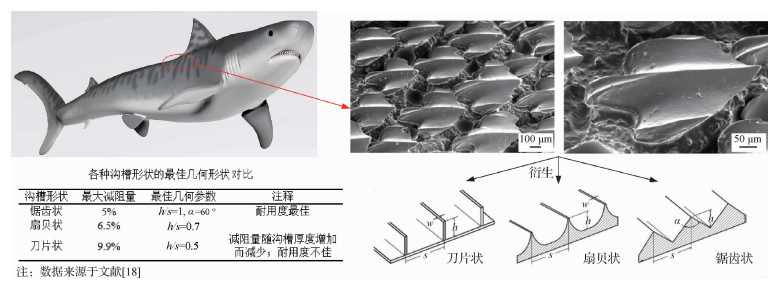图 1 鲨鱼皮衍生微观仿生表面参数化3D模型 Fig. 1 Parametric 3D model of micro-shark skin inspired structures bionic surface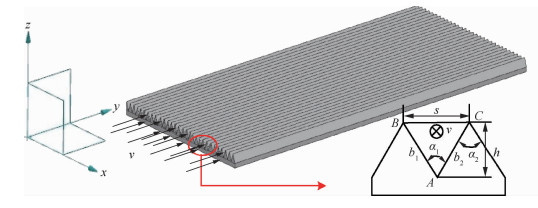图 2 微观仿生锯齿状表面示意图 Fig. 2 Schematic diagram of micro bionic zigzag surface

 $\left\{ {\begin{array}{*{20}{l}} {\frac{1}{2}{b_1}{b_2}{\rm{sin}}{\kern 1pt} {\kern 1pt} {\alpha _1} = \frac{1}{2}sh}\\ {b_1^2 + b_2^2 - 2{b_1}{b_2}{\rm{cos}}{\kern 1pt} {\kern 1pt} {\alpha _1} = {s^2}} \end{array}} \right.$

 $\left\{ {\begin{array}{*{20}{l}} {{b_1}{b_2}{\rm{sin}}{\kern 1pt} {\kern 1pt} {\alpha _1} - sh = 0}\\ {b_1^2 + b_2^2 - 2{b_1}{b_2}{\rm{cos}}{\kern 1pt} {\kern 1pt} {\alpha _1} - {s^2} = 0} \end{array}} \right.$

 $\left\{ {\begin{array}{*{20}{l}} {{b_1} = [ sh{\kern 1pt} {\kern 1pt} {\rm{cot}}{\alpha _1} + 0.5{s^2} - 0.5s({s^2} + 4{h^2} + }\\ {{\kern 1pt} {\kern 1pt} {\kern 1pt} {\kern 1pt} {\kern 1pt} {\kern 1pt} {\kern 1pt} {\kern 1pt} {\kern 1pt} {\kern 1pt} {\kern 1pt} {\kern 1pt} {\kern 1pt} {\kern 1pt} {\kern 1pt} {\kern 1pt} {\kern 1pt} {\kern 1pt} {\kern 1pt} 4hs{\kern 1pt} {\rm{cot}}{\alpha _1}{)^{0.5}}{]^{0.5}}}\\ {{b_2} = sh{\kern 1pt} {\kern 1pt} {\rm{csc}}{\alpha _1}[ sh{\kern 1pt} {\kern 1pt} {\rm{csc}}{\alpha _1} + 0.5{s^2} - 0.5s \cdot }\\ {{\kern 1pt} {\kern 1pt} {\kern 1pt} {\kern 1pt} {\kern 1pt} {\kern 1pt} {\kern 1pt} {\kern 1pt} {\kern 1pt} {\kern 1pt} {\kern 1pt} {\kern 1pt} {\kern 1pt} {\kern 1pt} {\kern 1pt} {\kern 1pt} {\kern 1pt} {\kern 1pt} {\kern 1pt} {{({s^2} - 4{h^2} + 4hs{\kern 1pt} {\rm{cot}}{\alpha _1})}^{0.5}}{]^{0.5}}} \end{array}} \right.$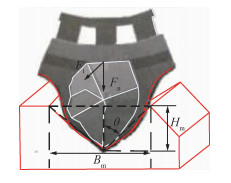图 3 砂带磨削单颗粒模型 Fig. 3 Single particle model for belt grinding

 ${A_{\rm{m}}}{(x)_{{\rm{max}}}} = \frac{1}{{\pi {\rm{tan}}{\kern 1pt} {\kern 1pt} \theta }}\left( {\frac{{3{F_{\rm{n}}}}}{{2mk{\kern 1pt} {\rm{HV}}}} - {A_{\rm{i}}}} \right)$ （1）

 ${H_{\rm{m}}} = \left( {\sqrt {\frac{{3{F_{\rm{n}}}}}{{2\pi mk{\kern 1pt} {\rm{HV}}}}} - \sqrt {\frac{{{A_{\rm{i}}}}}{\pi }} } \right){\rm{cot}}{\kern 1pt} {\kern 1pt} \theta$ （2）

 ${B_{\rm{m}}} = \sqrt {\frac{{6{F_{\rm{n}}}}}{{\pi mk{\kern 1pt} {\rm{HV}}}}}$ （3）

 ${H_{\rm{m}}} = \left( {\frac{{{B_{\rm{m}}}}}{2} - \sqrt {\frac{{{A_{\rm{i}}}}}{\pi }} } \right){\rm{cot}}\theta$ （4）

1.2 砂带磨削多颗粒磨削去除模型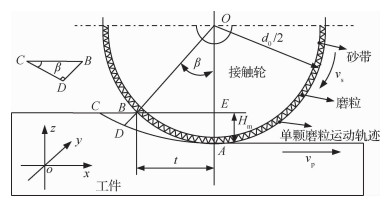图 4 砂带磨削多颗粒切削运动示意图 Fig. 4 Schematic diagram of belt grinding movement of multi particles

 ${h_{\rm{c}}} = BD/(\widehat {AB}{\kern 1pt} {b_{\rm{c}}}m)$ （5）

 $BD = BC{\kern 1pt} {\rm{sin}}{\kern 1pt} \beta$

 $\begin{array}{*{20}{l}} {{\rm{cos}}\beta = OE/OB = ({d_0}/2 - {H_{\rm{m}}})/({d_0}/2) = }\\ {{\kern 1pt} {\kern 1pt} {\kern 1pt} {\kern 1pt} {\kern 1pt} {\kern 1pt} {\kern 1pt} {\kern 1pt} {\kern 1pt} {\kern 1pt} {\kern 1pt} {\kern 1pt} {\kern 1pt} {\kern 1pt} {\kern 1pt} {\kern 1pt} {\kern 1pt} {\kern 1pt} {\kern 1pt} {\kern 1pt} {\kern 1pt} {\kern 1pt} {\kern 1pt} {\kern 1pt} {\kern 1pt} {\kern 1pt} ({d_0} - 2{H_{\rm{m}}})/{d_0}} \end{array}$

 ${\rm{sin}}\beta = \sqrt {1 - {\rm{co}}{{\rm{s}}^2}\beta } = 2\sqrt {{H_{\rm{m}}}/{d_0} - H_{\rm{m}}^2/d_0^2}$

 ${h_{\rm{c}}} = \frac{{2{v_{\rm{p}}}}}{{{v_{\rm{s}}}m{b_{\rm{c}}}}}\sqrt {{H_{\rm{m}}}/{d_0}}$ （6）

 ${H_{\rm{m}}} = \sqrt {\frac{{3{F_{\rm{n}}}}}{{2\pi mk{\kern 1pt} {\rm{HV}}}}} {\rm{cot}}\theta$ （7）

 ${h_{\rm{c}}} = {K_{\rm{c}}}{v_{\rm{p}}}v_{\rm{s}}^{ - 1}F_{\rm{n}}^{1/4}$ （8）

 ${K_{\rm{c}}} = {24^{1/4}}{\pi ^{ - 1/4}}b_{\rm{c}}^{ - 1}{m^{ - 5/4}}d_0^{ - 1/2}{k^{ - 1/4}}{\rm{H}}{{\rm{V}}^{ - 1/4}}{\rm{co}}{{\rm{t}}^{1/2}}\theta$ （9）

 $\left\{ {\begin{array}{*{20}{l}} {h = {h_{\rm{c}}} = {K_{\rm{c}}}{v_{\rm{p}}}v_{\rm{s}}^{ - 1}F_{\rm{n}}^{1/4}}\\ {{K_{\rm{c}}} = {{24}^{1/4}}{\pi ^{ - 1/4}}b_{\rm{c}}^{ - 1}{m^{ - 5/4}}d_0^{ - 1/2}{k^{ - 1/4}}{\rm{H}}{{\rm{V}}^{ - 1/4}}{\rm{co}}{{\rm{t}}^{1/2}}\theta }\\ {s = {B_{\rm{m}}} = \sqrt {\frac{{6{F_{\rm{n}}}}}{{\pi mk{\rm{HV}}}}} }\\ {{\alpha _1} = 2\theta } \end{array}} \right.$ （10）

2 微观仿生锯齿状表面磨削规划及其实验 2.1 叶片微观仿生锯齿状表面砂带磨削方法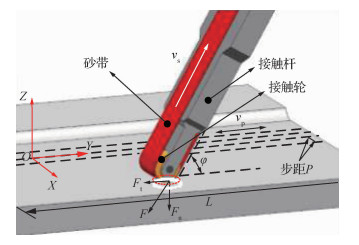图 5 微观仿生锯齿状表面砂带磨削方法 Fig. 5 Micro bionic zigzag surface using belt grinding method

 $\left\{ {\begin{array}{*{20}{l}} {T = {t_1} + {t_2} + {t_3}}\\ {{t_1} = \frac{{2L}}{{{v_{\rm{p}}}}},{t_2} = \frac{{2{h_1}}}{{{v_{\rm{步}}}}},{t_3} = \frac{P}{{{v_{\rm{步}}}}}} \end{array}} \right.$ （11）

2.2 实验装置及其方法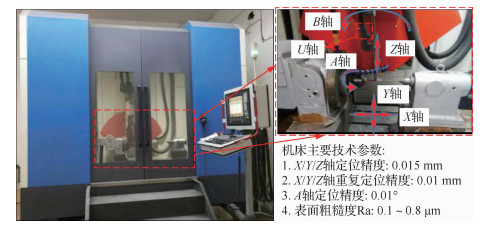图 6 MGY5540A-7NC数控砂带磨床 Fig. 6 MGY5540A-7NC CNC belt grinding machine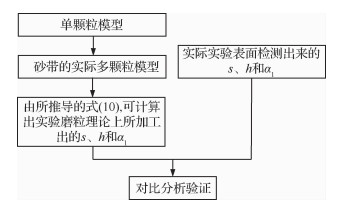图 7 研究思路流程 Fig. 7 Chart of research idea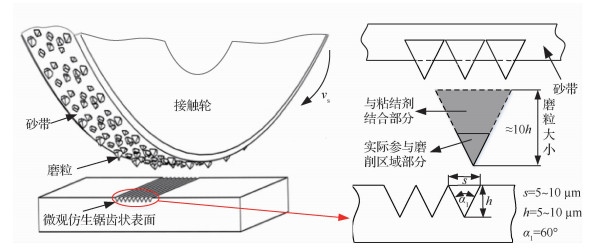图 8 砂带磨削微观仿生锯齿状表面示意图 Fig. 8 Schematic diagram of micro bionic zigzag surface using belt grinding

 磨削区域 磨削压力/N 进给速度/(m·s-1) 砂带线速度/(m·s-1) 进给步距/mm 型面 10 0.005 10 0.40
3 叶片微观仿生锯齿状表面磨削分析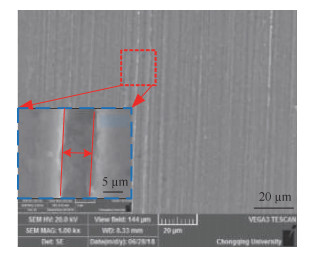图 9 磨削后微观仿生锯齿状SEM二维形貌表面 Fig. 9 SEM of two-dimensional morphology of micro bionic zigzag surface after belt grinding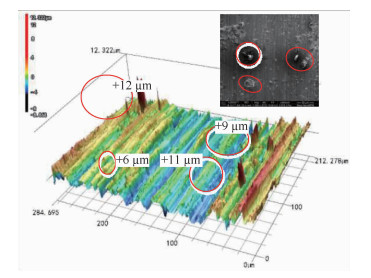图 10 砂带磨削前表面微观三维图 Fig. 10 Three-dimensional morphology surface before belt grinding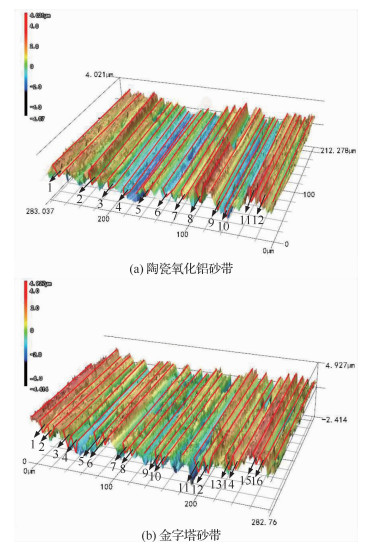图 11 砂带磨削后微观仿生锯齿状表面三维图 Fig. 11 Three dimensional morphology of micro bionic zigzag surface after belt grinding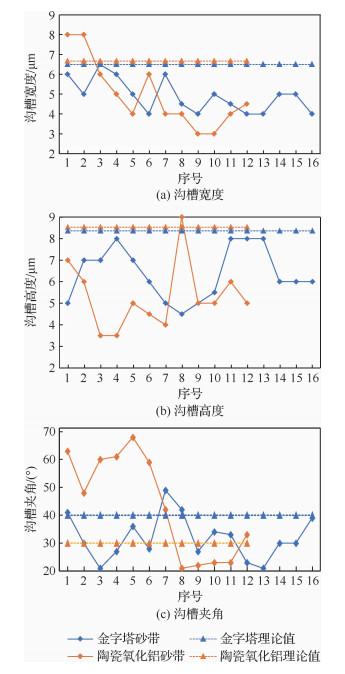图 12 两种砂带磨削表面参数对比 Fig. 12 Comparison of surface processed by two kinds of belt grinding parameters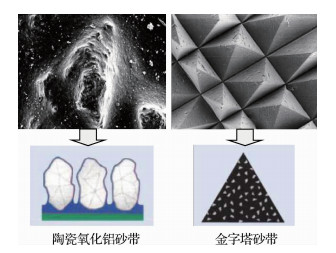图 13 两种砂带形貌示意图 Fig. 13 Schematic diagram of morphology of two kinds of belts

4 结论

1) 对微观仿生表面典型结构进行了分析，提出了将锯齿形沟槽结构作为叶片的微观仿生锯齿状表面。通过对单颗粒的砂带磨削去除机理的研究，建立了砂带磨削多颗粒参数化数学模型。最后，搭建了以钛合金为典型材料的微观仿生锯齿状表面砂带磨削基础实验平台。

2) 通过砂带磨削方法实现了微观仿生锯齿状表面的形成；砂带磨削后的叶片表面减少了凸凹不平和烧伤等缺陷，表面质量得到了提高；砂带表面磨粒分布情况能影响微观仿生锯齿状表面沟槽形成的大小和均匀性。对砂带磨削后的叶片表面进行检测，结果表明通过砂带磨削方法实现的微观仿生锯齿状表面以锯齿形沟槽为主。

  丁文锋, 奚欣欣, 占京华, 等. 航空发动机钛材料磨削技术研究现状及展望[J]. 航空学报, 2019, 40(6): 022763. DING W F, XI X X, ZHAN J H, et al. Research status and future development of grinding technology of titanium materials for aero-engine[J]. Acta Aeronautica et Astronautica Sinica, 2019, 40(6): 022763. (in Chinese) Cited By in Cnki (2) | Click to display the text  马付良, 曾志翔, 高义民, 等. 仿生表面减阻的研究现状与进展[J]. 中国表面工程, 2016, 29(1): 7-15. MA F L, ZENG Z X, GAO Y M, et al. Research status and progress of bionic surface drag reduction[J]. China Surface Engineering, 2016, 29(1): 7-15. (in Chinese) Cited By in Cnki (36) | Click to display the text  XIE J, XIE H F, LIU X R, et al. Dry micro-grooving on Si wafer using a coarse diamond grinding[J]. International Journal of Machine Tools and Manufacture, 2012, 61: 1-8. Click to display the text  ZHANG S J, ZHOU Y P, ZHANG H J, et al. Advances in ultra-precision machining of micro-structured functional surfaces and their typical applications[J]. International Journal of Machine Tools and Manufacture, 2019, 142: 16-41. Click to display the text  DENKENA B, KOEHLER J, WANG B. Manufacturing of functional riblet structure by profile grinding[J]. CIRP Journal of Manufacturing Science and Technology, 2010, 9(3): 14-26. Click to display the text  BRINKSMEIER E, SCHÖNEMANN L. Generation of discontinuous microstructures by diamond micro chiseling[J]. CIRP Annals-Manufacturing Technology, 2014, 63(1): 49-52. Click to display the text  高航, 李世宠, 付有志, 等. 金属增材制造格栅零件磨粒流抛光[J]. 航空学报, 2017, 38(10): 421210. GAO H, LI S C, FU Y Z, et al. Abrasive flow machining of additive manufactured metal grilling parts[J]. Acta Aeronautica et Astronautica Sinica, 2017, 38(10): 421210. (in Chinese) Cited By in Cnki (22) | Click to display the text  高航, 吴鸣宇, 付有志, 等. 流体磨料光整加工理论与技术的发展[J]. 机械工程学报, 2015, 51(7): 174-187. GAO H, WU M Y, FU Y Z, et al. Development of theory and technology in fluid abrasive fining technology[J]. Journal of Mechanical Engineering, 2015, 51(7): 174-178. (in Chinese) Cited By in Cnki (55) | Click to display the text  WANG W, YUN C. A path planning method for robotic belt surface grinding[J]. Chinese Journal of Aeronautics, 2011, 24(4): 520-526. Click to display the text  黄云, 肖贵坚, 邹莱. 整体叶盘抛光技术的研究现状及发展趋势[J]. 航空学报, 2016, 37(7): 2045-2064. HUANG Y, XIAO G J, ZOU L. Current situation and development trend of polishing technology for blisk[J]. Acta Aeronautica et Astronautica Sinica, 2016, 37(7): 2045-2064. (in Chinese) Cited By in Cnki (36) | Click to display the text  ECKART U, FLORIAN H. Improving efficiency in robot assisted belt grinding of high performance materials[J]. Advanced Materials Research, 2014, 907: 139-149. Click to display the text  ECKART U, FLORIAN H, MARCEL M, et al. Applicability of industrial robots for machining and repair processes[J]. Procedia CIRP, 2013, 11: 234-238. Click to display the text  XU X H, ZHU D H, ZHANG H Y, et al. Application of novel force control strategies to enhance robotic abrasive belt grinding quality of aero-engine blades[J]. Chinese Journal of Aeronautics, 2019, 32(10): 2368-2382. Click to display the text  张雷, 周宛松, 卢磊, 等. 抛光力实时控制策略研究[J]. 东北大学学报(自然科学版), 2015, 36(6): 853-857. ZHANG L, ZHOU W S, LU L, et al. Research on real-time control strategies of polishing force[J]. Journal of Northeastern University (Nature Science), 2015, 36(6): 853-857. (in Chinese) Cited By in Cnki (4) | Click to display the text  肖贵坚, 黄云, 尹浩. 面向型面精度一致性的整体叶盘砂带磨削新方法及实验研究[J]. 航空学报, 2016, 37(5): 1666-1676. XIAO G J, HUANG Y, YIN H. Experimental research of new belt grinding method for consistency of blisk profile and surface precision[J]. Acta Aeronautica et Astronautica Sinica, 2016, 37(5): 1666-1676. (in Chinese) Cited By in Cnki (18) | Click to display the text  XIAO G J, HUANG Y. Equivalent self-adaptive belt grinding for the real-R edge of an aero-engine precision-forged blade[J]. The International Journal of Advanced Manufacturing Technology, 2016, 83(9-12): 1697-1706. Click to display the text  蔺小军, 杨艳, 吴广, 等. 面向叶片型面的五轴联动柔性数控砂带抛光技术[J]. 航空学报, 2015, 36(6): 2074-2082. LIN X J, YANG Y, WU G, et al. Flexible polishing technology of five-axis NC abrasive belt for blade surface[J]. Acta Aeronautica et Astronautica Sinica, 2015, 36(6): 2074-2082. (in Chinese) Cited By in Cnki (25) | Click to display the text  BECHERT D W, BRUSE M, HAGE W, et al. Experiments on drag reducing surfaces and their optimization with an adjustable geometry[J]. Journal of Fluid Mechanics, 1997, 338: 59-87. Click to display the text  XIAO G J, HE Y, HUANG Y, et al. Shark-skin-inspired micro-riblets forming mechanism of TC17 titanium alloy with belt grinding[J]. IEEE Access, 2019, 7(1): 107636-107648. Click to display the text  CLAUDIA C B, SCHULZ U. Shark skin inspired riblet structures as aerodynamically optimized high temperature coatings for blades of aeroengines[J]. Smart Materials & Structures, 2011, 20(9): 094016.
http://dx.doi.org/10.7527/S1000-6893.2019.23288

0

#### 文章信息

XIAO Guijian, HE Yi, HUANG Yun, LI Wei, LI Quan

Single particle removal model and experimental study on micro bionic zigzag surface of aeronautical blade using belt grinding

Acta Aeronautica et Astronautica Sinica, 2020, 41(7): 623288.
http://dx.doi.org/10.7527/S1000-6893.2019.23288